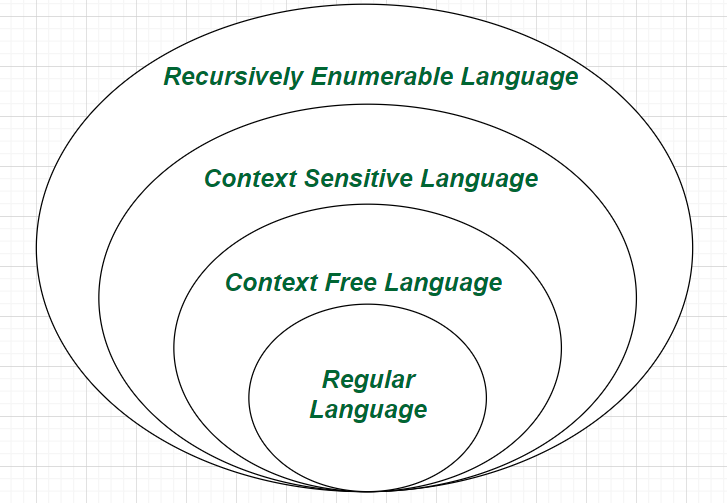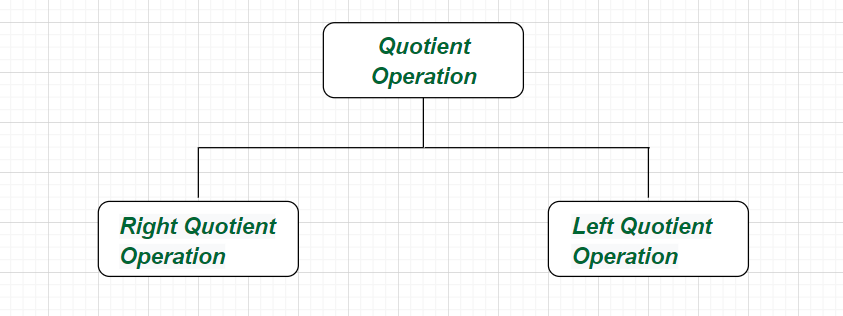# Quotient Operation in Automata

• Difficulty Level : Medium
• Last Updated : 29 Jun, 2021

Overview :
According to the theoretical aspects of Automata, a quotient operation can be defined as the technique that recognizes a superset of the given automation particularly for the given string of formal language (A formal language is a set of strings of symbols drawn from a finite alphabet. It can have rules based on regular expressions or a context free grammar that generates/accepts the language). So, a quotient automata can be obtained from a given non-deterministic finite automations by joining some of its states.

Closure properties of the quotient operation :
Some common and important closure properties of the quotient operation in theory of Automata are as follows.

• The quotient operation of a regular language with any other language is regular.
• The quotient operation of a context free language with a regular language is context free.
• Quotient operation of two recursively enumerable languages is recursively enumerable.
• The quotient operation two context free languages can be any recursively enumerable language.Types of Quotient operation :
The Quotient operation can be divided into two parts as follows.Type-1 :
Right Quotient (Cutting in Right) –

` L1/L2 = { a | ab ∈  L1 for some b ∈  L2 }                                 `

Here, ∑ (a, b). The given expression states the right quotient operation of language L1 with respect to L2 which is language consisting of strings ‘b’ such that ‘ab’ is in L1 for some string ‘b’ in L2.

Example-1 :

```Let   L1 = { hooray, sunray, defray, ray},
L2 = { ray}
so,   L1/L2 = { hoo , sun, def,  ∈ } ```

Example-2 :

```Let   L1 = {∈, a, ab, aba, abab, ... },
L2 = { b, bb, bbb, bbbb, ... }
so,   L1/L2 = { a, aba, ababa, ... } ```

Type-2 :
Left Quotient (Cutting in Left) –

`L1/L2 = { b | ab ∈  L1 for some a ∈  L2 }                                `

Here, ∑ (a, b). The given expression states the left quotient operation of language L1 with respect to L2 which is language consisting of strings ‘a’ such that ‘ab’ is in L1 for some string ‘a’ in L2.

Example-1 :

```Let   L1 = { match, matter, mat, matzoth },
L2 = { mat}
so,   L1/L2 = { tch , ter, ∈ , zoth } ```

Example-2 :

```Let   L1 = { 10, 100, 1010, 101110},
L2 = { 10}
so,   L1/L2 = { ∈ , 0, 10, 1110 } ```
My Personal Notes arrow_drop_up
Recommended Articles
Page :